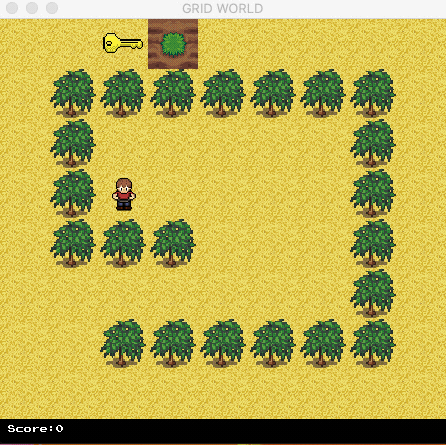## Monte-Carlo Method

In this tutorial, we will explain how to create a new RL algorithm (Monte-Carlo) in FruitAPI. By the use of FruitAPI, a Monte-Carlo (MC) learner can be created under 50 lines of code. Basically, the MC method generates as many as possible the number of episodes. In each episode, it saves the agent's states, actions, and rewards. When the episode ends, it calculates the return value of every state-action pairs. The action-value function is the average of all return values of different episodes. In this way, the policy converges to the optimal solution.

Moreover, we recognize that Monte-Carlo and Q-Learning are quite similar. Q-Learning updates the value function during the episode while Monte-Carlo waits until an episode ends. Therefore, we can inherit a Q-Learning learner and modify its behavior via the function `update()`. This call-back function will be notified in every step. Therefore, users do not need to create episodes and manage them. The source code can be found here `/fruit/learners/mc.py`.

After creating an MC learner, we can plug it into the framework and train the agent. The following code explains this:

``````def train_mc_grid_world():
engine = GridWorld(render=False, graphical_state=False, stage=1,
number_of_rows=8, number_of_columns=9, speed=1000,
seed=100, agent_start_x=2, agent_start_y=2)

environment = FruitEnvironment(game_engine=engine)

agent = AgentFactory.create(MCLearner, network=None, environment=environment,
checkpoint_frequency=1e5,
num_of_epochs=1, steps_per_epoch=1e5,
learner_report_frequency=10,
log_dir='./train/grid_world/mc_checkpoints')

agent.train()```
```

The framework frequenly saves the value function into `log_dir` in every `checkpoint_frequency` steps. After training, we can load a checkpoint and evaluate it as follows.

``````def eval_mc_grid_world():
engine = GridWorld(render=True, graphical_state=False, stage=1,
number_of_rows=8, number_of_columns=9, speed=2,
seed=100, agent_start_x=2, agent_start_y=2)

environment = FruitEnvironment(game_engine=engine)

agent = AgentFactory.create(MCLearner, network=None, environment=environment,
checkpoint_frequency=1e5,
num_of_epochs=1, steps_per_epoch=1e4,
learner_report_frequency=50,
log_dir='./test/grid_world/mc_checkpoints',
When testing, we should specify `epsilon_annealing_start=0` or the agents will move randomly. The testing source code can be found here `/fruit/samples/mc_test.py`.Contact us at `hello@fruitlab.org` or join our community at `https://www.facebook.com/groups/fruitlab/` to ask any questions.